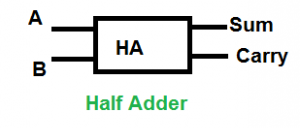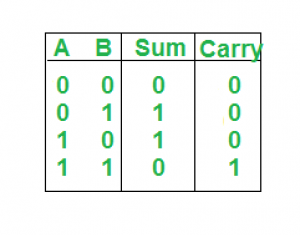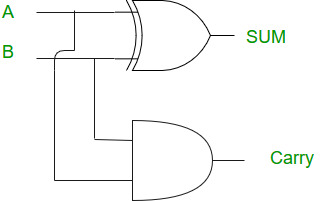Related Articles

# Half Adder in Digital Logic

• Difficulty Level : Easy
• Last Updated : 25 Sep, 2020

The addition of 2 bits is done using a combination circuit called Half adder. The input variables are augend and addend bits and output variables are sum & carry bits. A and B are the two input bits.Truth Table:Logical Expression:
Sum = A XOR B
Carry = A AND B

Implementation: